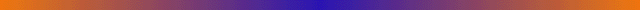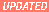ChaosBook.org | projects | webbook help | Cvitanović travel ]

GEOMETRY OF CHAOS

## "Statistical Physics Out of Equilibrium", Inst. Henri Poincaré, Paris, 10 lectures October 22-26 2007 - Lecture notes

You might want to download and read the chapters in advance of the lectures, skipping the sections indicated. Chapters here are currently more up-to-date than on ChaosBook.org1. A brief history of motion in time
An overview of the course. Skim quickly through - parts will make sense only in the rear view mirror, do not worry that you do not understand them yet.
Appendix A. Brief history of chaos (optional reading) Classical mechanics has not stood still since Newton. The formalism that we use today was developed by Euler and Lagrange. By the end of the 1800's the three problems that would lead to the notion of chaotic dynamics were already known: the three-body problem, the ergodic hypothesis, and nonlinear oscillators.
2. Trajectories
A recapitulation of basic notions of dynamics. The reader familiar with the dynamics on the level of an introductory graduate nonlinear dynamics course can safely skip this material, hop to chapter 14: Transporting densities. J
3. Maps
Discrete time dynamics arises by considering sections of a continuous flow. There are also many settings in which dynamics is discrete, and naturally described by repeated applications of a map.
4. Stability
Review of basic concepts of local dynamics: local linear stability for flows and maps. [skip section 4.4.1]
5. Cycle stabilities are invariant
Cycle eigenvalues are flow invariants. [skip sections 5.3, 5.4 and 5.5]
6. Billiards
7. Cycling for (discrete) fundamentalists
8. Partitioning state space
Qualitative dynamics of simple stretching and mixing flows; Smale horseshoes and symbolic dynamics. The topological dynamics is incoded by means of transition matrices/Markov graphs. [skip sections 10.1.1 and 10.5]
10. Markov graphs
12. Fixed points: where are they?
13. Chaos for accountants
Topological zeta function; determinants of graphs; fear not infinite partitions
You learn here how to count and describe itineraries. While computing the topological entropy from transition matrices/Markov graphs, we encounter our first zeta function. [skip sections 13.4.1 and 13.5]
16. Learning how to measure
A first attempt to move the whole phase space around - natural measure and fancy operators. [skip section 14.5]
17. Not your average theory
On the necessity of studying the averages of observables in chaotic dynamics. Formulas for averages are cast in a multiplicative form that motivates the introduction of evolution operators. [skip sections 15.3 and 15.4]
18. All that was left was a trace
If there is one idea that one should learn about chaotic dynamics, it happens in this chapter: the (global) spectrum of the evolution is dual to the (local) spectrum of periodic orbits. The duality is made precise by means of trace formulas. [skip sections 14.2.1, 14.3 and 14.4]
20. Grimly determined
We derive the spectral determinants, dynamical zeta functions. [skip sections 15.2, 15.3.1, 15.3.2, 15.5 and 15.6]
22. Recycle: it's the law
Spectral eigenvalues and dynamical averages are computed by expanding spectral determinants into cycle expansions, expansions ordered by the topological lengths of periodic orbits. [skip sections 18.1.3, 18.1.4, 18.2.2, 18.2.4, 18.4 and 18.5]
24. Cycling for (smooth) fundamentalists
25. Get used to it
In the preceding chapters we have moved at rather brisk pace and derived a gaggle of formulas. Here we slow down in order to develop some fingertip feeling for the objects derived so far. Just to make sure that the key message - the ``trace formulas'' and their ilk - have sunk in, we rederive them in a rather different, more intuitive way, and extol their virtues. This part is bedtime reading. A few special determinants are worked out by hand. [skip sections 20.1.1, 20.1.2, 20.3 and 20.4]svn: \$Author: predrag \$ - \$Date: 2007-10-19 07:29:33 -0400 (Fri, 19 Oct 2007) \$